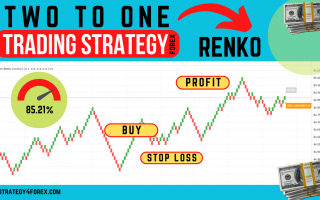# RENKO Forex strategy «Two To One»

Forex strategy «Two to One» is based on the RENKO chart, on this site we have already considered the strategy based on the RENKO chart «FXR — Modern». But unlike her, the trading system, which we will consider in this article, does not use any indicators and is simple to the point of «disgrace».

First, we need to get a RENKO chart in the MT4 terminal using the RenkoExpertAdvisor auxiliary expert (this process is described in detail in the article for the «FXR — Modern» strategy ➜).

Some statistics:

The key to the profitability of this strategy lies in the observance of the ratio of profit to loss on each transaction 2/1. Due to this, the strategy has made a profit since the beginning of May in the amount of 560 points (by 4 digits). There are 69 trades with a positive outcome, 82 trades with a negative outcome. But despite the large number of losing trades, the profit is still greater!

If you look closely at the chart, you will find that many bricks have vertical lines in the opposite direction. This line is called the «wick». It is known that according to the RENKO chart, in order to change the direction of building bricks, the price needs to go in the opposite direction a distance equal to two brick sizes. If it fails and the price continues to move in the previous direction, then a wick appears on the chart the size of the distance that the price traveled when trying to change direction.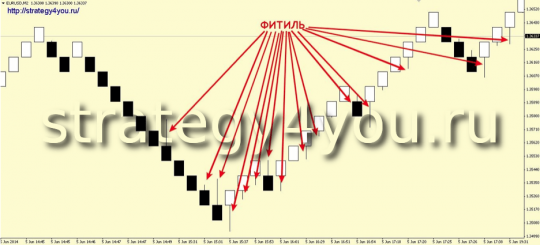The brick size is 10 points.

The currency pair is recommended EUR/USD. For other pairs, you should independently choose the size of the brick.

## Conditions for PURCHASES under the Forex Strategy «Two to One»: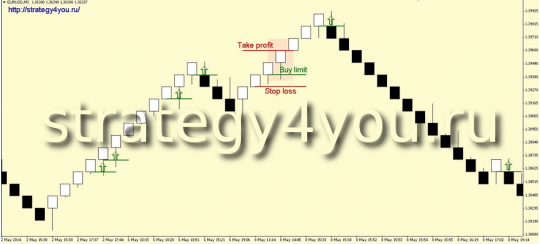1) The previous brick must be white (bullish).

2) The price drops to the opening level of the previous brick (in our case, it drops by 10 points).

3) It is at this level that we should open a long position (you can also use a Buy Limit order).

4) Stop loss is equal to the size of the brick, that is, 10 points.

5) Take profit is equal to the size of a brick multiplied by two, that is, 20 points.

## Conditions for SALES under the «Two for One» Strategy: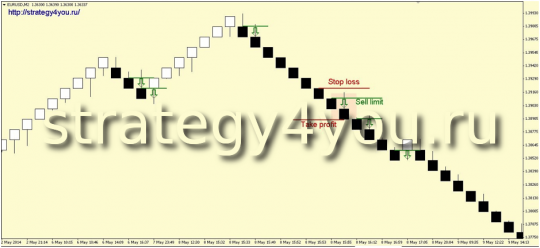1) The previous brick must be black (bearish).

2) The price rises to the opening level of the previous brick (in our case it rises by 10 points).

3) It is at this level that we should open a short position (you can also use a Sell Limit order).

4) Stop loss is equal to the size of the brick, that is, 10 points.

5) Take profit is equal to the size of a brick multiplied by two, that is, 20 points.

For clarity, see the following figure:

Green ticks indicate deals with a positive outcome (9 pcs), and red crosses — deals with a negative outcome (12 pcs).

9 x 20 = 180 points

12 x 10 = 120 points.

180 – 120 = 60 pips profit.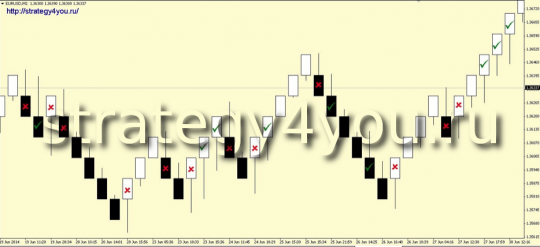### Video Forex Strategy «Two to One»:

original